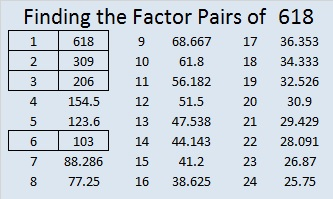# 618 and Level 3

618 is even so it is obviously divisible by 2. Is it divisible by 3?

Yes, 618 has the same digits as 16 & 8. Since 16 is 8 doubled, 618 is divisible by 3.

618  is the sum of consecutive prime numbers 307 and 311.

618 can be written as the sum of 4 consecutive numbers because 618 is greater than 6 and is divisible by 2, but not by 4. Thus 153 + 154 + 155 + 156 = 618.Print the puzzles or type the solution on this excel file: 10 Factors 2015-09-14

—————————————————————————————————

• 618 is a composite number.
• Prime factorization: 618 = 2 x 3 x 103
• The exponents in the prime factorization are 1, 1, and 1. Adding one to each and multiplying we get (1 + 1)(1 + 1)(1 + 1) = 2 x 2 x 2 = 8. Therefore 618 has exactly 8 factors.
• Factors of 618: 1, 2, 3, 6, 103, 206, 309, 618
• Factor pairs: 618 = 1 x 618, 2 x 309, 3 x 206, or 6 x 103
• 618 has no square factors that allow its square root to be simplified. √618 ≈ 24.8596.—————————————————————————————————

A Logical Approach to solve a FIND THE FACTORS puzzle: Find the column or row with two clues and find their common factor. (None of the factors are greater than 10.)  Write the corresponding factors in the factor column (1st column) and factor row (top row).  Because this is a level three puzzle, you have now written a factor at the top of the factor column. Continue to work from the top of the factor column to the bottom, finding factors and filling in the factor column and the factor row one cell at a time as you go.